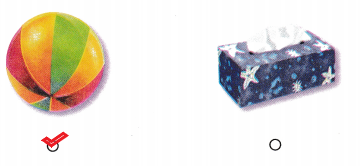# Texas Go Math Kindergarten Lesson 18.3 Answer Key Identify Spheres

Refer to our Texas Go Math Kindergarten Answer Key Pdf to score good marks in the exams. Test yourself by practicing the problems from Texas Go Math Kindergarten Lesson 18.3 Answer Key Identify Spheres.

## Texas Go Math Kindergarten Lesson 18.3 Answer Key Identify Spheres

Essential Question
How do you sort, classify, and identify spheres?

Explore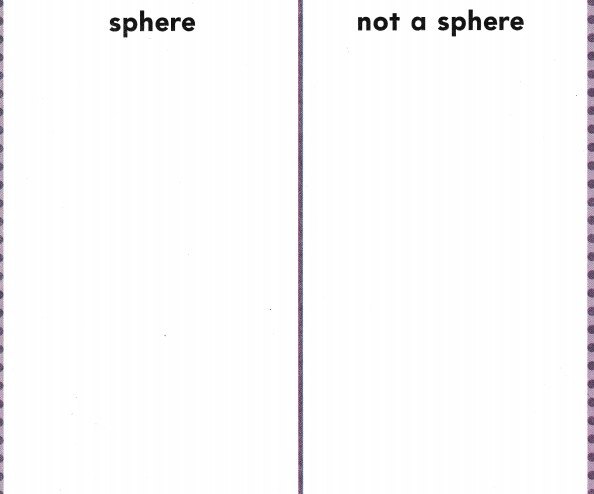Explanation:
I drew a sphere and i drew some shapes that are not spheres.

Directions
Use three-dimensional shapes and identify the sphere. Sort the shapes. Describe the sphere. Match a picture of each shape to the shapes on the sorting mat. Glue the shape pictures to show how you sorted.

Share and Show

Question 1.Explanation:
A sphere has a curved surface
So, i drew a circle around the words that describe a sphere.

Question 2.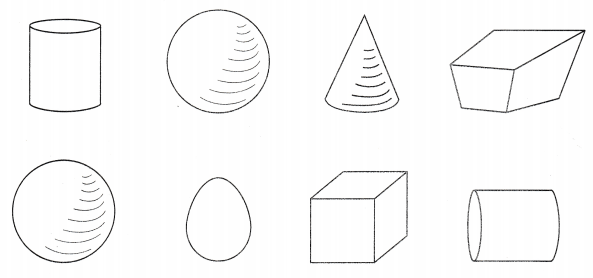Explanation:
I colored the spheres.

Directions
1. Look at the sphere. Circle the words that describe a sphere. 2. Color the spheres.

Question 3.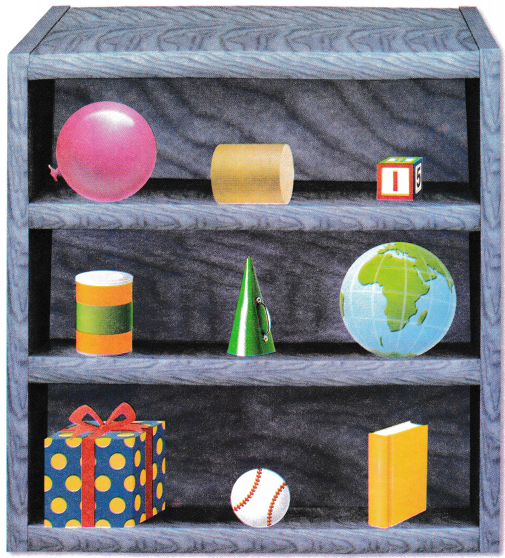Explanation:
I identified the objects that are shaped like a sphere and marked an X on those objects.

Directions
3. Identify the objects that are shaped like a sphere. Mark an X on those objects.

Home Activity

• Have your child identify and describe a household object that is shaped like a sphere.

Problem Solving

Question 4.Explanation:
A sphere has a curved surface
So, i marked X on the sphere.

Question 5.Explanation:
I checked the object that is not shaped like a sphere.

Directions
4. I have a curved surface. Which shape am I? Mark on X on that shape. 5. Choose the correct answer. Which shape is not a sphere?

### Texas Go Math Kindergarten Lesson 18.3 Answer Key Identify Cubes

Question 1.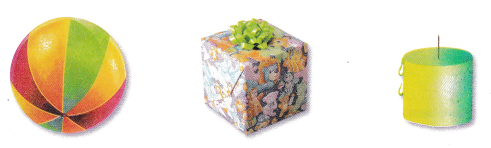Explanation:
I drew a circle around the object that is shaped like a sphere and marked on X on the objects that are not shaped like a sphere.

Question 2.Explanation:
I drew a circle around the object that is shaped like a sphere and marked on X on the objects that are not shaped like a sphere.

Question 3.Explanation:
I drew a circle around the object that is shaped like a sphere and marked on X on the objects that are not shaped like a sphere.

Directions
1-3. Sort the shapes. Circle the object that is shaped like a sphere. Mark on X on the objects that are not shaped like a sphere.

Texas Test Prep

Lesson Check

Question 4.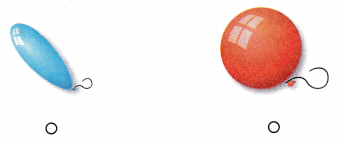Explanation:
I checked the red balloon
It is shaped like a sphere.

Question 5.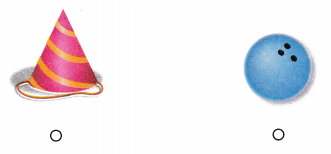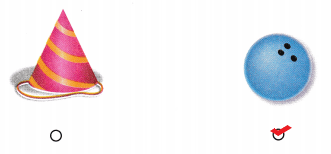Explanation:
I checked the object that is shaped like a sphere.

Question 6.